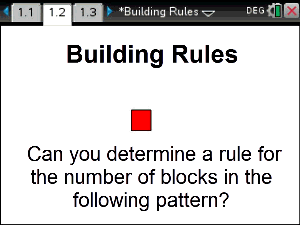# Activities

•• ##### Subject Area

• Standard: VCE: Mathematical Methods: Mathematical Methods

• ##### AuthorAust Senior

30 Minutes

• ##### Device
• TI-Nspire™ CAS
• TI-Nspire™ Navigator™
• ##### Software

TI-Nspire™ CAS

4.0

## Building Rules#### Activity Overview

This activity explores three different ways to generate a rule for a particular interactive and dynamic block pattern. Students use symbolic notation to develop algebraic expressions and represent functions, solve systems of simultaneous equations and produce graphs from data. At the end of the activity students are provided with a powerful visual representation of the same problem that helps them produce the same equation simply and easily.

#### Objectives

Find the equation of a quadratic given sufficient information (ACMMM009)

#### Vocabulary

Polynomial, degree of polynomial, quadratic, difference table